Courses
Courses for Kids
Free study material
Free LIVE classes
More

# Complex Numbers Concept of Rotation for JEELIVE
Join Vedantu’s FREE Mastercalss

## What is Complex Number?

Being an extension of the real number system, complex numbers have numerous interesting properties that make mathematical calculations even easier. These useful properties model two-dimensional space and trigonometry. A complex number is a point on the complex or argand plane with the horizontal component as the real part and the vertical component as the imaginary part. Take for example z= 2 + 6i. Here, 2 is the horizontal component and 6 is the vertical component.

Besides the several useful properties of complex numbers, one might wonder what is the rotation of complex numbers. Rotation of complex numbers is an easier and streamlined way to correlate the complex numbers and the angle that they make.

## What is Rotation?

The relationship between the complex numbers and the angle that they make is referred to as the rotation of a complex number. A complex rotation can be denoted as $|z| {e}^{i \theta}$ where θ is a real number in radians. ∅ denotes the rotation of the complex number in an anticlockwise or counterclockwise direction and -θ for the clockwise direction.

Let us understand this concept thoroughly.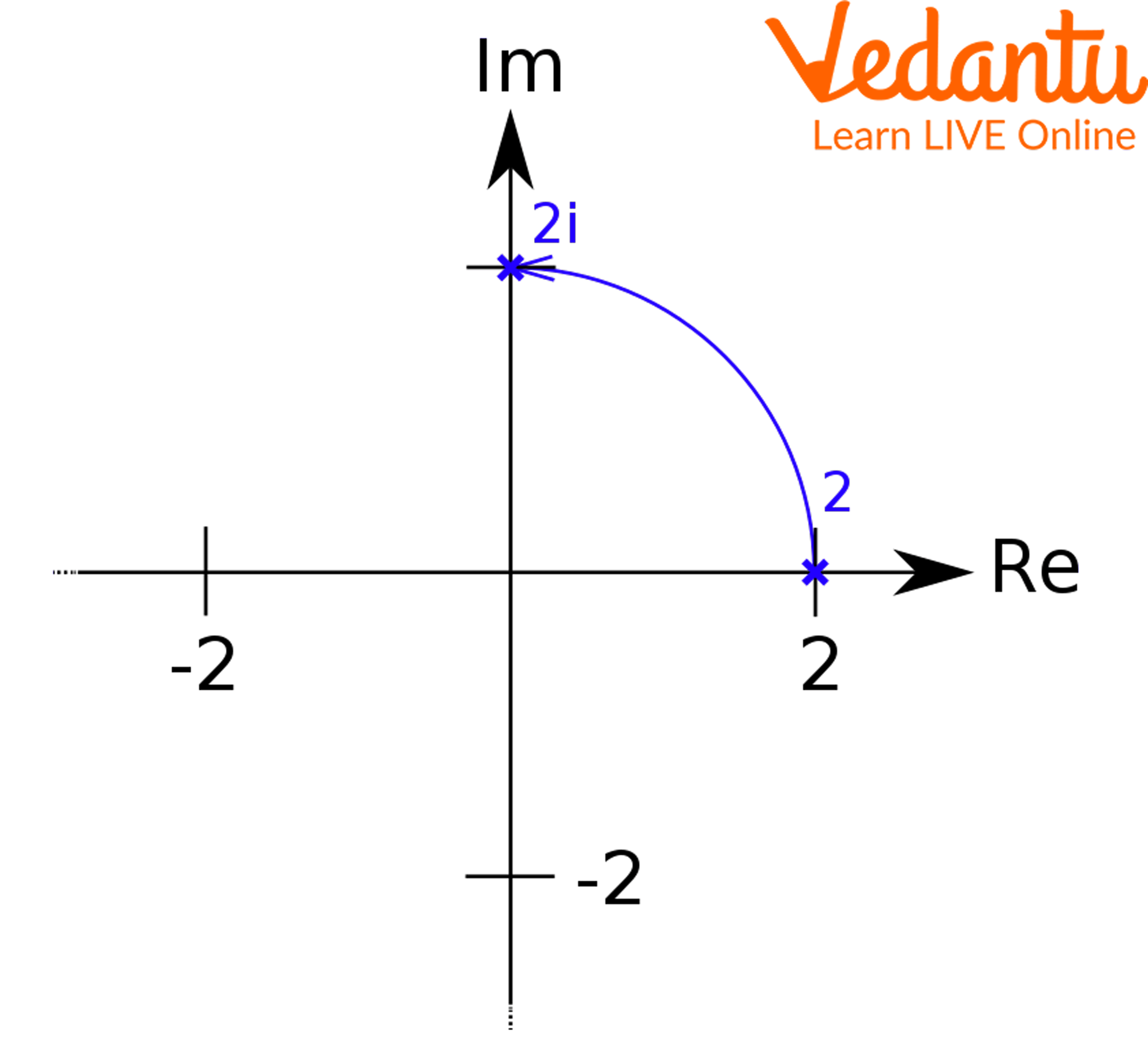Concept of Rotation

## Rotation of Complex Numbers

Take another example, in the figure below, there are two complex numbers z and z’. We want to rotate oz’ along with oz. It can be achieved when we rotate the complex number by the argument of $\dfrac{Z}{Z^{\prime}}$.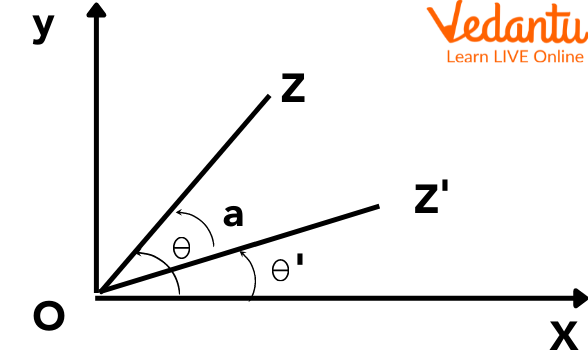Rotation of a Complex Number

Further,

$\dfrac{z}{z^{\prime}}=\dfrac{\left|z_{r}\right| e^{i \theta}}{\left|z^{\prime}\right| e^{i θ^{\prime}}} \\ \dfrac{z}{z^{\prime}}=\dfrac{|z|}{\left|z^{\prime}\right|} e^{i \alpha x}$

Or

$\dfrac{z}{z^{\prime}}=\dfrac{|z|}{\left|z^{\prime}\right|}(\cos (\alpha)+i \sin (\alpha))$

The above concept can also be looked at this way.

$z=|z| e^{i \theta}$-----(1)

And

$z^{\prime}=\left|z^{\prime}\right| e^{i \theta^{\prime}}$------(2)

By dividing (1) and (2), we get

$\dfrac{z}{z^{\prime}}=\frac{|z|}{\left|z^{\prime}\right|} e^{i\left(\theta-\theta^{\prime}\right)} \\ \dfrac{z}{z^{\prime}}=\dfrac{|z|}{\left|z^{\prime}\right|} e^{i \alpha}$

It is the same result that we got above. Thus, from the above results, we conclude that to rotate the complex number, we can use $\dfrac{z}{z^{\prime}}=\dfrac{|z|}{\left|z^{\prime}\right|} e^{i \alpha}$ result directly.

## Solved Examples for Rotation of Complex Numbers

Question 1: With its origin at the centre, consider an n-sided polygon. If z1 is the complex number representing a vertex B1 of the polygon, then find the complex number associated with the vertex adjacent to B1, i.e, m B2 and Bn.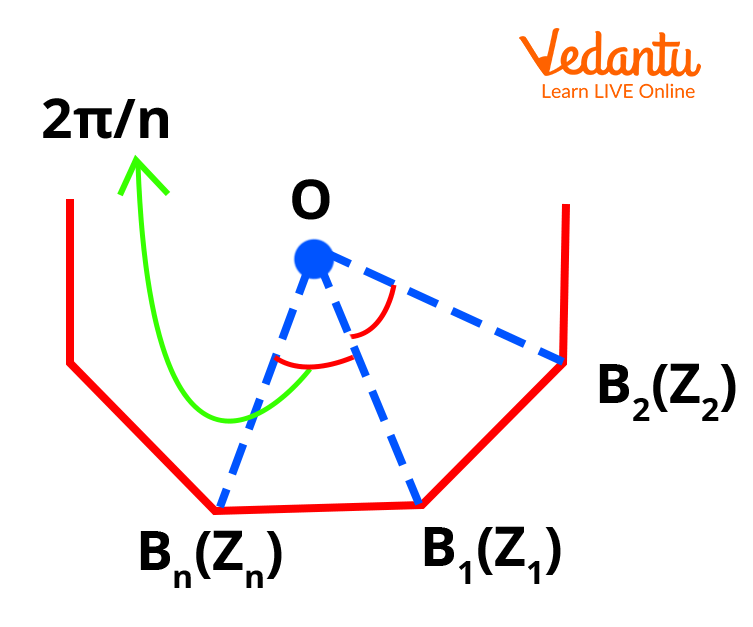N-Sided-Polygon

Solution: From the figure above, we know that the vertex adjacent to B1 is either B2 or Bn.

Now we know from above that

$\dfrac{z}{z^{\prime}}=\dfrac{|z|}{\left|z^{\prime}\right|} e^{i \alpha}$

Using the same relationship,

$\dfrac{z_{2}-0}{z_{1}-0}=\dfrac{\left|z_{2}\right|}{\left|z_{1}\right|} e^{\dfrac{i n}{\pi}} \\ z_{2}=z_{1} e^{\dfrac{i n}{\pi}} \\ \dfrac{z_{n}-0}{z_{1}-0}=\dfrac{\left|z_{n}\right|}{\left|z_{1}\right|} e^{\dfrac{-i n}{\pi}} \\ \\ n=z_{1} e^{\dfrac{-i n}{\pi}}$

Question 2: Complex numbers z1, z2, and z3 are the vertices P, Q, and R, respectively, of an isosceles right-angled triangle with a right angle at R . Show that (z1 – z2)2 = 2(z1 – z3)(z3 – z2).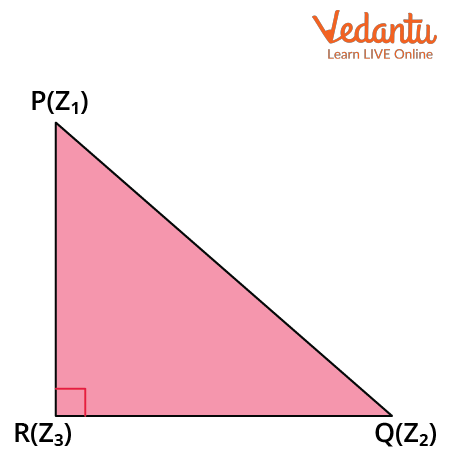Rotation of a Complex Number

Solution: In the isosceles triangle PQR, PQ = QR and PQ are at 90 degrees to QR which means that QR has to be rotated to occupy the position PQ.

$\dfrac{z_{2}-z_{3}}{z_{1}-z_{3}}=e^{\dfrac{i \pi}{2}}=i \\ z_{2}-z_{3}=i\left(z_{1}-z_{3}\right)$

On Squaring and solving, we get the result

z22 + z32 – 2 z2 z3 = -(z12 + z32– 2 z1 z3)

(z1 – z2)2 = 2(z1 – z3)(z3 – z2).

Question 3: Rotate the complex number (5+6i) using the multiplicative property of complex numbers.

Solution: Multiply, the above complex number (5+6i) with i. It will then be

$(5+6 i) \times i=5 i+(6 i) \times i \\ (5+6 i) i=5 i+6 \times(-1) \\ (5+6 i) i=-6+5 i$

This gives us the result (5+6i).i = -6+5i.

## Rotation Number

Multiplication of a complex number with i. The rotation property of a complex number can also be correlated with the multiplication property of the complex number. It means that when we multiply a complex number with iota or i of magnitude 1, we find that it leads to a pure rotation of the complex number. Now, this rotation could also be extended to 180, 270 degrees, and so on.

Look at the below diagram. Here, the complex number (4+3i) is multiplied by i to give it a rotation of 90 degrees in the complex plane.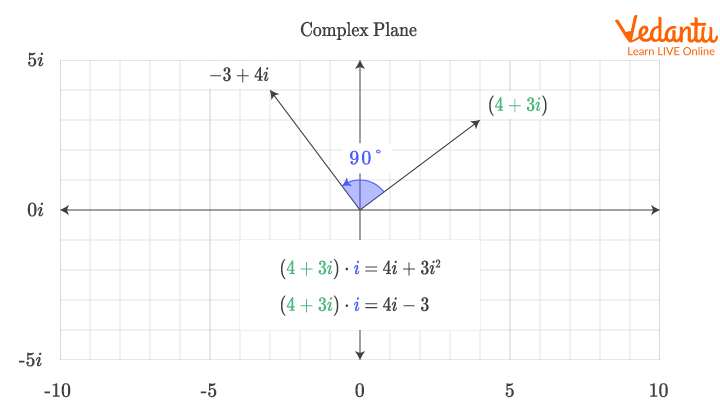Complex Number Rotation

## Rotation Theorem in Complex Numbers

The rotation theorem of the complex theorem is as follows:

• If P(z1) and Q(z2) are two complex numbers such that |z1| = |z2|, then $z^{2}=z_{1}^{\text {ei } \theta}$, where $\theta$ is the argument between z1 and z2.

• If P(z1), Q(z2), and R(z3) are three complex numbers and θ is the angle between z1, z2, and z3, then

$\dfrac{z_{3-} z_{2}}{z_{1}-z_{2}}=\left|\dfrac{z_{3}-z_{2}}{z_{1}-z_{2}}\right| e^{i \theta}$

• If P(z1), Q(z2), R(z3), and S(z4) are three complex numbers and θ is the angle between the z1, z2, z3, and z4, then

$\dfrac{z_{3}-z_{4}}{z_{1}-z_{2}}=\left|\dfrac{z_{3}-z_{4}}{z_{1}-z_{2}}\right| e^{i \theta}$

$\dfrac{z_{2}-z_{1}}{z_{4}-z_{3}}$

Other things to note are:

• amp (z) = $\theta$ is a line from the origin inclined at an angle from the x-axis.

• Multiplication by $e^{-I\alpha}$ to z rotates the vector in clockwise direction by an angle $\alpha$.

• If z1, z2, z3, and z4 are the vectors defining the points A, B, C, and D, respectively, in the Argand plane, then

• AB is inclined to CD at an angle= arg $\dfrac{z_{2}-z_{1}}{z_{4}-z_{3}}$.

• If CD is inclined at 90o to AB, then arg $=\dfrac{z_{2}-z_{1}}{z_{4}-z_{3}}=\pm \dfrac{\pi}{2}$

## Conclusion

The article traverses through the concepts of rotation of complex numbers. Rotation of complex numbers with reference to multiplication property and the different theorems are listed. The theorems provide an understanding of how the complex numbers rotate on the argand plane, with some particular angle. They also illustrate the rotation of different vectors on the argand plane with respect to one another. It is also seen that on multiplying a complex number with i, it leads to its rotation by 90 degrees. There is a pure rotation as the multiplication factor is i, whose magnitude is 1.

Last updated date: 21st Sep 2023
Total views: 130.8k
Views today: 2.30k

## FAQs on Complex Numbers Concept of Rotation for JEE

1. Is the rotation of complex numbers related to complex multiplication?

Yes, the rotation of a complex number can directly be correlated with the multiplication property. In such a case, the rotation property can be denoted as (x+iy)i.

Multiplying a complex number by i represents that the complex number is rotated by 90 degrees in the anticlockwise or counterclockwise direction.  Thus, rotation in a complex plane can be visualised as stretching and rotating. The reason this multiplication results in a pure rotation and no stretching is because we are multiplying the complex number by one. This rotation property of the complex number can be extended to common rotations like 0, 90, 180, and 270 degrees.

2. What is a unimodular complex number?

A unimodular complex number is a complex number with a unit magnitude.

Suppose z is a complex number. Thus, the unimodular complex number is represented by |z|=1. Since |z|=1, it means z lies on a circle of radius 1 and with a centre (0,0).

Take for example, z1 and z2 are complex numbers such that $\dfrac{z_{1}-z_{2}}{2-z_{1} \overline{z_{2}}}$ is unimodular and z2 is not unimodular.

To solve the above problem, we take $\left|\dfrac{z_{1}-z_{2}}{4-z_{1} \overline{z_{2}}}\right|=1$. On solving, we get the value of z1 as a circle of radius 2.